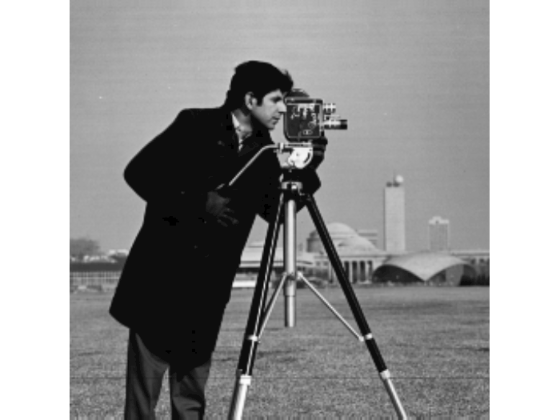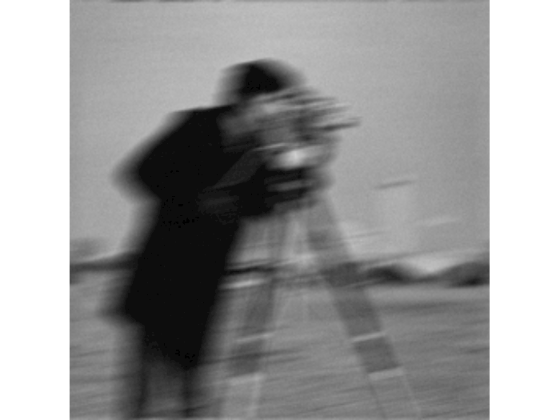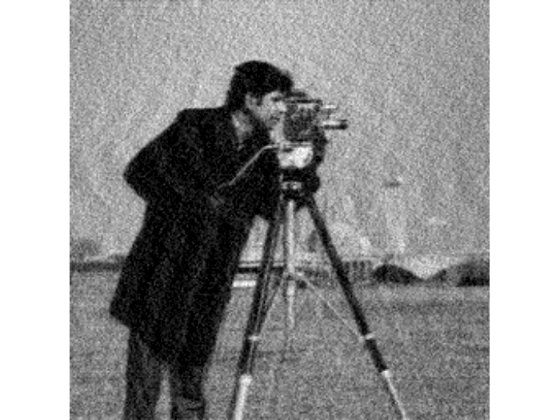Documentation

deconvwnr

Deblur image using Wiener filter

Description

example

J = deconvwnr(I,psf,nsr) deconvolves image I using the Wiener filter algorithm, returning deblurred image J. psf is the point-spread function (PSF) with which I was convolved. nsr is the noise-to-signal power ratio of the additive noise. The algorithm is optimal in a sense of least mean square error between the estimated and the true images.

J = deconvwnr(I,psf,ncorr,icorr) deconvolves image I, where ncorr is the autocorrelation function of the noise and icorr is the autocorrelation function of the original image.

J = deconvwnr(I,psf) deconvolves image I using the Wiener filter algorithm with no estimated noise. In the absence of noise, a Wiener filter is equivalent to an ideal inverse filter.

Examples

collapse all

Read image into the workspace and display it.

imshow(I);
title('Original Image (courtesy of MIT)');Simulate a motion blur.

LEN = 21;
THETA = 11;
PSF = fspecial('motion', LEN, THETA);
blurred = imfilter(I, PSF, 'conv', 'circular');
figure, imshow(blurred)noise_mean = 0;
noise_var = 0.0001;
blurred_noisy = imnoise(blurred, 'gaussian', ...
noise_mean, noise_var);
figure, imshow(blurred_noisy)
title('Simulate Blur and Noise')Try restoration assuming no noise.

estimated_nsr = 0;
wnr2 = deconvwnr(blurred_noisy, PSF, estimated_nsr);
figure, imshow(wnr2)
title('Restoration of Blurred, Noisy Image Using NSR = 0')Try restoration using a better estimate of the noise-to-signal-power ratio.

estimated_nsr = noise_var / var(I(:));
wnr3 = deconvwnr(blurred_noisy, PSF, estimated_nsr);
figure, imshow(wnr3)
title('Restoration of Blurred, Noisy Image Using Estimated NSR');Input Arguments

collapse all

Blurry image, specified as a numeric array of any dimension.

Data Types: single | double | int16 | uint8 | uint16

Point-spread function, specified as a numeric array.

Data Types: double

Noise-to-signal ratio, specified as a nonnegative scalar or numeric array of the same size as the image, I. If nsr is an array, then it represents the spectral domain. Specifying 0 for the nsr is equivalent to creating an ideal inverse filter.

Data Types: double

Autocorrelation function of the noise, specified as a numeric array of any size or dimension, not exceeding the original image.

• If the dimensionality of ncorr matches the dimensionality of the image I, then the values correspond to the autocorrelation within each dimension.

• If ncorr is a vector and psf is also a vector, then the values in ncorr represent the autocorrelation function in the first dimension.

• If ncorr is a vector and psf is an array, then the 1-D autocorrelation function is extrapolated by symmetry to all non-singleton dimensions of psf.

• If ncorr is a scalar, then the value represents the power of the image noise.

Data Types: double

Autocorrelation function of the image, specified as a numeric array of any size or dimension, not exceeding the original image.

• If the dimensionality of icorr matches the dimensionality of the image I, then the values correspond to the autocorrelation within each dimension.

• If icorr is a vector and psf is also a vector, then the values in icorr represent the autocorrelation function in the first dimension.

• If icorr is a vector and psf is an array, then the 1-D autocorrelation function is extrapolated by symmetry to all non-singleton dimensions of psf.

• If icorr is a scalar, then the value represents the power of the image noise.

Data Types: double

Output Arguments

collapse all

Deblurred image, returned as a numeric array. J has the same data type as I.

Tips

• The output image J could exhibit ringing introduced by the discrete Fourier transform used in the algorithm. To reduce the ringing, use I = edgetaper(I,psf) before calling deconvwnr.

 Gonzalez, R. C., and R. E. Woods. Digital Image Processing. Addison-Wesley Publishing Company, Inc., 1992.